02 4651 0000 [email protected]
/ / How to Estimate Turf Quantities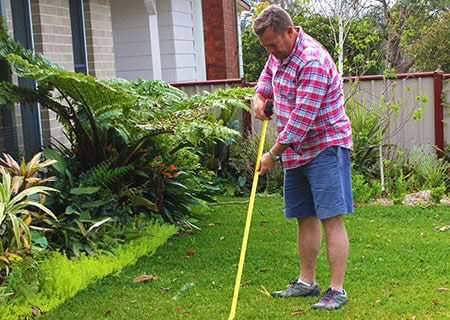# How to Estimate Turf Quantities

Dec 13, 2018

Simply break the area up into basic shapes and dust off your geometry formulas!

To measure the area of your new lawn, simply create a sketch, break it up into simple shapes, calculate the areas of each of these shapes and add them all together to find the total area.## 1. Create a sketch

Create a sketch of the area you plan to turf, remember to include any paths, gardens, pools, and so on.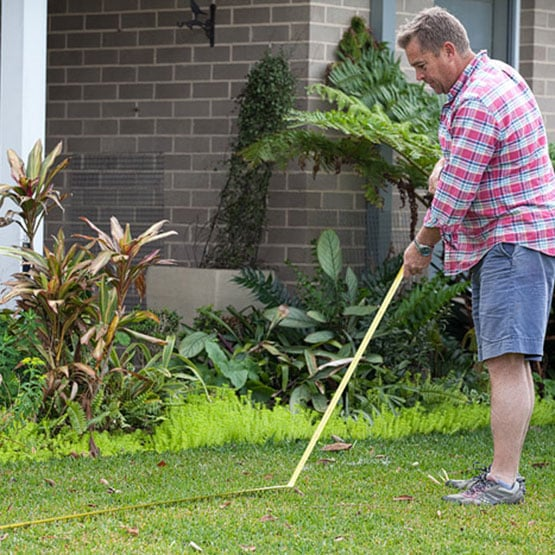## 2. Establish Simpler Shapes

If needed, break the sketch up into simpler shapes, such as circles, rectangles and triangles, to make the calculations easier.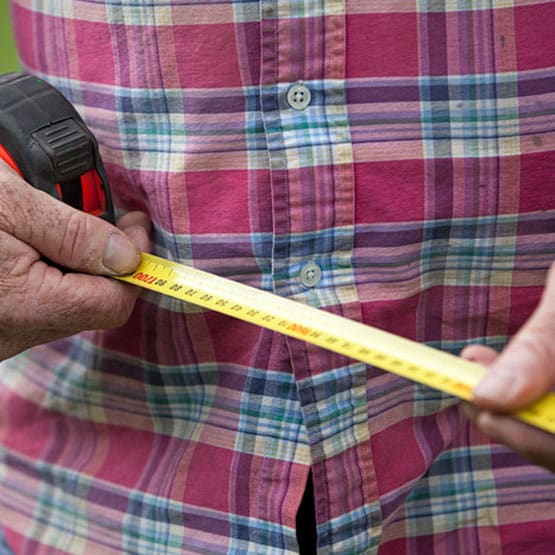## 3. Measure the areas

Using a tape measure, record the actual dimensions of each shape in your sketch. Calculate the area of the shapes, and then add all the areas together to get a total.

Make sure you add a few extra rolls to your order to allow for falling short and for off-cuts.## 4. How Do I find the Area of Different Shapes?

For the following calculations, you should use metres (m) for all your measurements, this will ensure your totals are in metres, and will make it easier to calculate how many rolls of turf you will need.

To calculate the area of a rectangle, simply find the width and height, and multiply them together. The area of a rectangle = w x h

To calculate the area of a triangle, find the width and height, multiply them together and divide by 2. The area of a triangle = (w x h)/2

To calculate the area of a circle, find the diameter, divide it by 2, square that and multiply by pi (3.14). The area of a circle = 3.14 x (d/2)2

For a semi-circle, use the same as a circle, but divide area by 2 – area of a semi-circle = (3.14 x (d/2)2)/2

To find the overall area of a more complex shape, add together individual areas of the various shapes.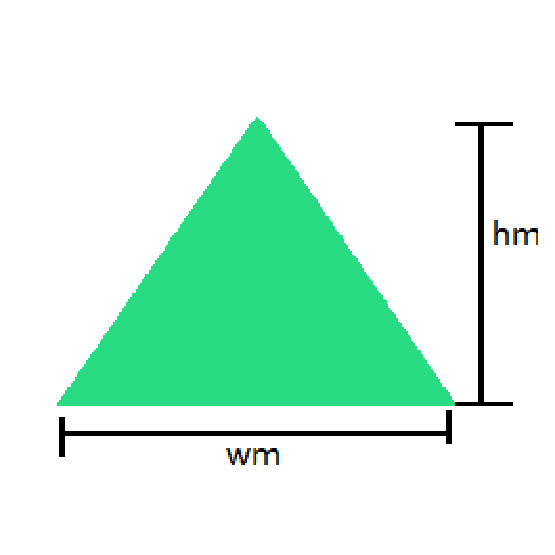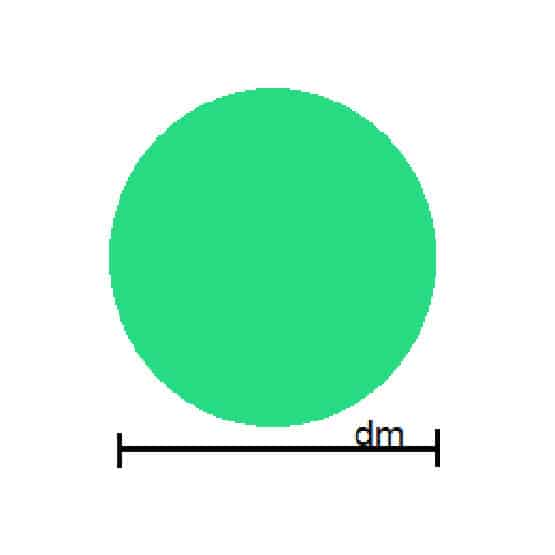## 5. Calculate How Many Rolls You Need

Each turf roll is equivalent to about 1m2, measuring 1.72 metres long and 0.58cm wide.

The depth, depending on the grass species chosen, ranges from 0.25mm to 0.30mm.

## Turf Calculator

 Shape Width (m) Height (m) Total (m2) Please select Rectangle Triangle Circle Semicircle
Total (m2)